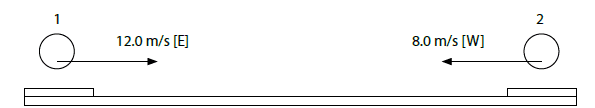# Electric Potential Energy & Electric Potential

student07
Two frictionless pucks are placed on a level surface, as shown, at an initial distance of 20 m. The mass of puck 1 is 0.80 kg and it has a charge of +3.0 x 10^-4 C, while puck 2 is 0.40 kg with a charge of +3.0 x 10^-4 C. The initial velocity of puck 1 is 12 m/s [E] and the initial velocity of puck 2 is 8 m/s [W]. Find the minimum separation of the two pucks (the minimum distance between the pucks).Attempt at the solution:
Let right be positive
Kq1q2/r = 0.5 -m1v1^2 + m2v2^2
9 x 10^9 Nm^2/C^2(3 x 10 ^-4 C) ( 3 x 10^-4 C) /r = 0.5(-0.80)(-12)^2 + (0.40)(8)^2
810Nm^2 / r = 70.4 kgm^2/s^2
r = 11.51m
20 - 11.51 = 8.50m

Is this right?

## Answers and Replies

nasu
They have PE in both states: initial and final.
And the way you write it, seems that you have 1/2 only for one of the kinetic energies.

student07
.5 (0.80) (12)^2 = 57.6, .5(0.40)(8)^2 = 12.8
57.6 + 12.8 = 70.4 same results

nasu
I don't know what these numbers mean.
But the conservation of energy is missing the initial PE.
Write the equations first and then start with the numbers.

How can be 70 m when they approach each other from 20 m?

Last edited:
Chris Jarvis
Not to take away from the OP's questions but I'm on this question as we speak; If we use the conservation of momentum, wouldn't the two pucks final velocity be equal to zero at the closest point? Since like charges repel, would their closest point of contact not be when all the energy is in the form of electric potential energy; i.e. v2f=0? so wouldn't the equation to solve this just be:
1/2m1v1o+1/2m2v2o+kq1q2/ro =0+kq1q2/rf?

Gold Member
wouldn't the two pucks final velocity be equal to zero at the closest point?
Their speeds would be maximum but, throughout the process, the total Momentum would be the same as at the start. When they are very close, the value of the Potential will be governed by their actual radii, so the question is not fully specified.
And the way you write it, seems that you have 1/2 only for one of the kinetic energies
It's because he hasn't used parentheses properly in the first formula. Not such a problem when one's using a pencil and paper. AS nasu says, it's best to stick wih the algebra right up until the end and then stick in the numbers. The pattern of the symbols makes it easier to spot mistakes.

•Chris Jarvis